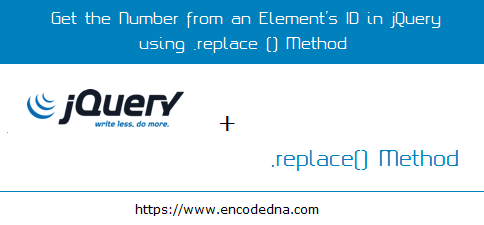﻿ How to Get the Number from an Element’s ID using jQuery replace() Method Example

# How to Get the Number from an Element’s ID using jQuery replace() Method Example

Here I am sharing a simple tip on how to get the number from an HTML element’s ID using jQuery. The element that you use can be a DIV or an input box. Now, sometimes we might add numbers (numeric values) to the element’s id to make the ids look unique. You can have other reasons too.Let us assume, I have dynamically created few DIV elements, using either a server side script or JavaScript, and each element has a unique id, prefixed with a number sequence. I wish to get the number (only) from each element’s id, when clicked.

The Markup
```<!DOCTYPE html>
<html>
<title>Get the Number or Numeric Value from an Element's ID</title>
<body>
<p>Click the Elements below to get the number from its ID.</p>

<div id="div1">
Contents in DIV1
</div>
<div id="div2">
Contents in DIV2
</div>

<p id="result"></p>
</body>

<script>
\$('div').click(function () {
var i = \$(this).attr('id').replace('div', '');
\$('#result').text(i);
});
});
</script>
</html>```
In the above script, I am using two different methods to extract the number from each element. The first method is .attr(), which takes a parameter as id. The second method is .replace(), which takes two parameters, the string value we want to replace followed by the value to replace.

The second parameter in the .replace() is blank. This gives us the number replacing the value div.

## Second Example

Here’s another example. This might be useful tool. I have a table. Each row has an Input box (textbox), and two DIV elements. Each element has a unique id prefixed by a number. The first field (input box) with accept a number as Quantity. The second is a DIV element, which has a predefined value as Price. Once I enter value in the “Quantity” field, my jQuery script will calculate Quantity and Price and show the total in the last DIV element of the row.

The Code
```<!DOCTYPE html>
<html>
<body>
<style>
div{
display:inline-table;
margin:2px 5px;
}
input {
width:100px;
color:#333;
}
</style>

<p>Enter any number in the first (Quantity) field. It will calculate the Quantity, multiplied by the Price and show the Total Amount.</p>

<div>
<div><input placeholder="Qty." id="qty1" /></div>
x <div id="price1">2000</div>
= <div id="total1"></div><br />
<div><input placeholder="Qty." id="qty2" /></div>
x <div id="price2">1600</div>
= <div id="total2"></div><br />
<div><input placeholder="Qty." id="qty3" /></div>
x <div id="price3">2100</div>
= <div id="total3"></div>
</div>
</body>

<script>